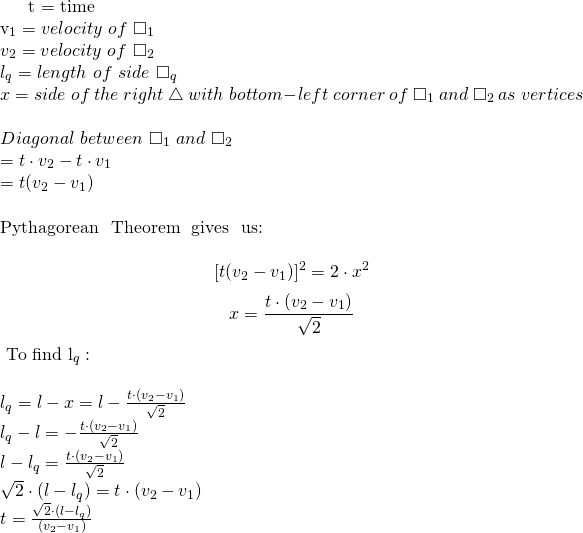# Solution: HackerRank Sherlock and Moving Tiles in Ruby

This one was pretty fun! To be honest, I found it a little tricky at first but once I got the hang of it the math was pretty easy. The key is understanding that the velocity of each square is how much they each move on the line y = x every interval. NOT how much to increment each (x,y) coordinate every interval as I naively thought.

So, first of all, let’s check out the question prompt and get a solid grasp of the question. Then let’s do some math to solve for t, which is the amount of time needed for square q to be the proper area.Apologies for the LaTeX with the poor formatting, I’m not entirely used to it yet. Perhaps with a few more of these problems I will be!

Anyway, when we solve for time we’re basically looking for when one side of square q will be the square root of the sought-after area. In order to implement this in Ruby I built a simple PORO that just implements the equation from above in Ruby.

Remember, though, that the velocity of square 1 could be larger than the velocity of square 2. The equation above was built with the velocity of square 2 being greater than the velocity of square 1. There are a couple ways to account for this, but I simply just took the absolute value of the result to achieve the correct answer.

This worked for every test case. Were you able to solve this problem? What strategies did you use to do so?